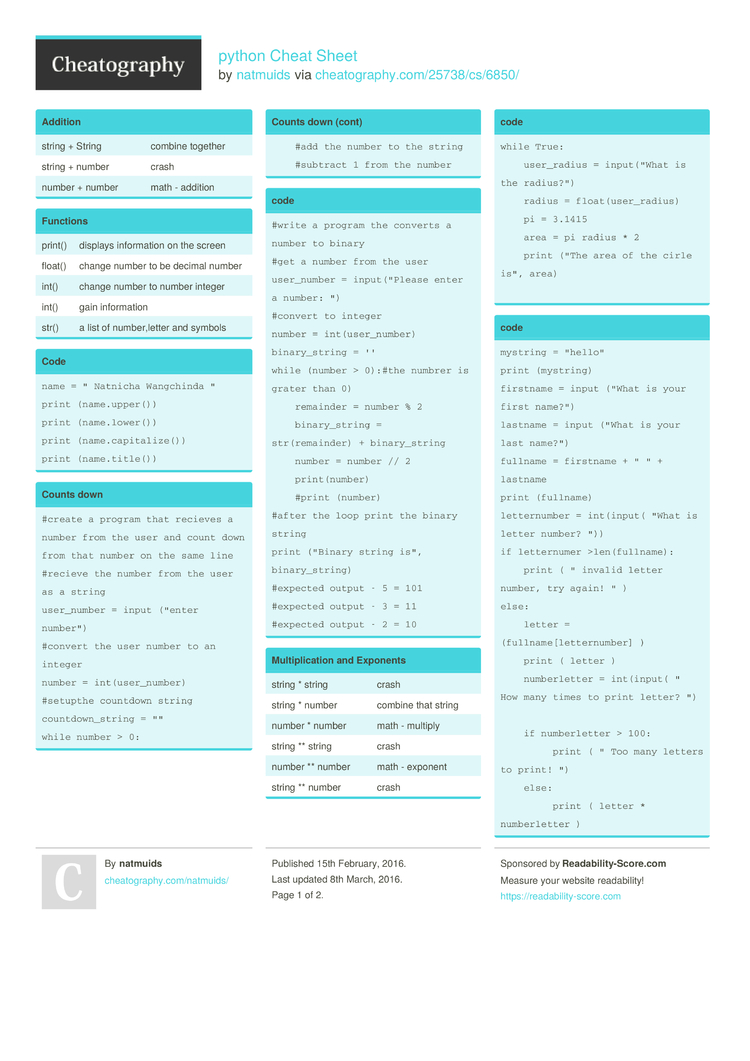# python Cheat Sheet by natmuids

 string + String combine together string + number crash number + number math - addition

### Functions

 print() displays inform­ation on the screen float() change number to be decimal number int() change number to number integer int() gain inform­ation str() a list of number­,letter and symbols

### Code

 ```name = " Natnicha Wangchinda " print (name.upper()) print (name.lower()) print (name.capitalize()) print (name.title())```

### Counts down

 ```#create a program that recieves a number from the user and count down from that number on the same line #recieve the number from the user as a string user_number = input ("enter number") #convert the user number to an integer number = int(user_number) #setupthe countdown string countdown_string = "" while number > 0:     #add the number to the string     #subtract 1 from the number```

### code

 ```#write a program the converts a number to binary #get a number from the user user_number = input("Please enter a number: ") #convert to integer number = int(user_number) binary_string = '' while (number > 0):#the numbrer is grater than 0)     remainder = number % 2     binary_string = str(remainder) + binary_string     number = number // 2     print(number)     #print (number) #after the loop print the binary string print ("Binary string is", binary_string) #expected output - 5 = 101 #expected output - 3 = 11 #expected output - 2 = 10```

### Multip­lic­ation and Exponents

 string * string crash string * number combine that string number * number math - multiply string ** string crash number ** number math - exponent string ** number crash

### code

 ```while True:     user_radius = input("What is the radius?")     radius = float(user_radius)     pi = 3.1415     area = pi radius * 2     print ("The area of the cirle is", area)```

### code

 ```mystring = "hello" print (mystring) firstname = input ("What is your first name?") lastname = input ("What is your last name?") fullname = firstname + " " + lastname print (fullname) letternumber = int(input( "What is letter number? ")) if letternumer >len(fullname):     print ( " invalid letter number, try again! " ) else:     letter = (fullname[letternumber] )     print ( letter )     numberletter = int(input( " How many times to print letter? ")                             if numberletter > 100:          print ( " Too many letters to print! ")     else:          print ( letter * numberletter )```

### code

 ```def createList(quitword):     mylist = []     while True :         item = input("Please enter a list item: ")         if (item == quitword) :             return (mylist)         duplicateword = False         for myvar in mylist:             if myvar == item:                 duplicateword = True         if duplicateword == True :             print('Duplicate word!')         else:             mylist.append (item) mylist = createList("stop") print (mylist)```

### Vocabulary

 Variable hold a value and can be change string a list of character such as number­,le­tter, and symbols integer number whole number or counting number float number the number in decimal syntax gramma or structure of language value the number os string can be store in valuable module the text for storing for python code input gain inform­ation print to show inform­ation on the screen syntax error make impossible to the parse error

### code

 ```# Create a program that recieve a number from the user # from that number on the same line #recieve the number from the user as a string user_number = input #convert the user number to an integer number = int(user_number) #setup the countdown string countdown_string = "" while number > ():#the number is greater than 0)     remainder =     print (number)     #binary_string = #output should look like this # if the user enters 5: # 5 4 3 2 1 print (countdown_string)```

### Math

 + plus - minus * multiple / divide % remainder (4%2)-> 0 ** exponent 2**3->2^3 == equal to != no equal to < less than > more than <= less than or equal to >= more than or equal to2 Pages
//media.cheatography.com/storage/thumb/natmuids_python.750.jpg

PDF (recommended)